Samacheer Kalvi 10th Maths Solutions Chapter 2 Numbers and Sequences Ex 2.7

Tamilnadu Samacheer Kalvi 10th Maths Solutions Chapter 2 Numbers and Sequences Ex 2.7

Ex 2.7 Class 10 Samacheer Question 1.
Which of the following sequences are in G.P?
(i) 3, 9, 27, 81, ……..
(ii) 4, 44, 444, 4444, ………
(iii) 0.5, 0.05, 0.005, ……..
(iv) $$\frac { 1 }{ 3 }$$,$$\frac { 1 }{ 6 }$$,$$\frac { 1 }{ 12 }$$………
(v) 1, -5, 25, -125, …….
(vi) 120, 60, 30, 18, …….
(vii) 16, 4, 1, $$\frac { 1 }{ 4 }$$, ……
Solution:
(i) 3, 9, 27, 81
r = Common ratio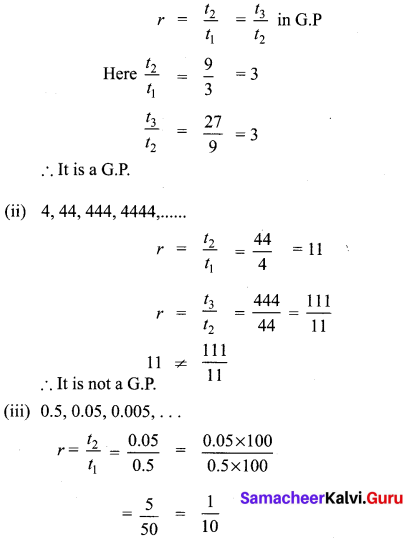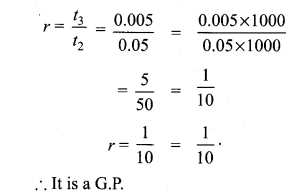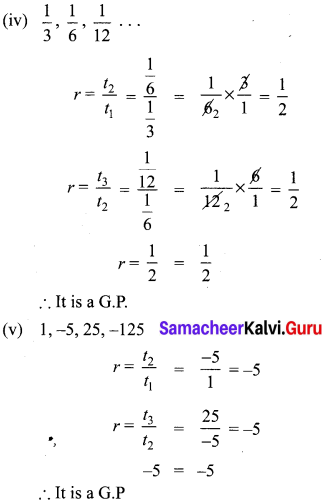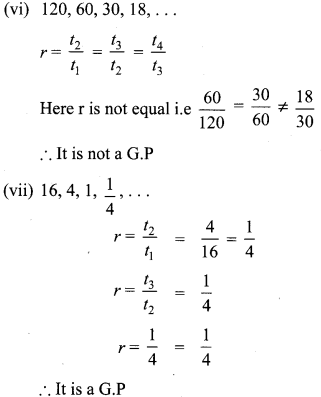10th Maths Exercise 2.7 Samacheer Kalvi Question 2.
Write the first three terms of the G.P. whose first term and the common ratio are given below.
(i) a = 6, r = 3
(ii) a = $$\sqrt { 2 }$$ , r = $$\sqrt { 2 }$$
(iii) a = 1000, r = $$\frac { 2 }{ 5 }$$
Solution:
(i) a = 6, r = 3
tn = arn-1
t1 = ar1-1 = ar0 = a = 6
t2 = ar2-1 = ar1 = 6 × 3 = 18
t3 = ar3-1 = ar2 = 6 × 32 = 54
∴ The 3 terms are 6, 18, 54, ….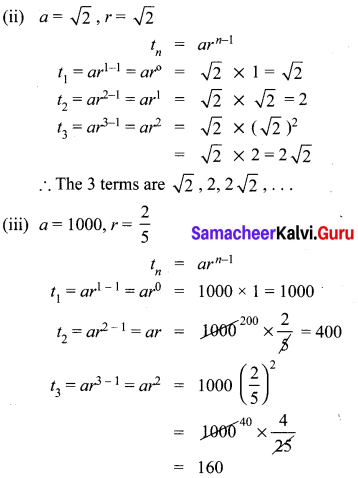The 3 terms are 1000, 400, 160, ……………

10th Maths Exercise 2.7 Question 3.
In a G.P. 729, 243, 81,… find t7.
Solution:
G.P = 729, 243, 81 ……
t7 = ?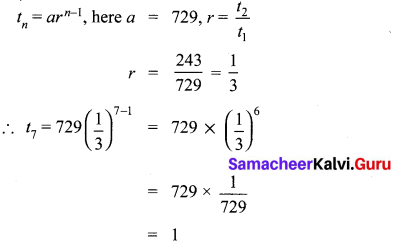Exercise 2.7 Class 10 Maths Question 4.
Find x so that x + 6, x + 12 and x + 15 are consecutive terms of a Geometric Progression.
$$\frac{t_{2}}{t_{1}}$$ = $$\frac { x+12 }{ x+6 }$$,$$\frac{t_{3}}{t_{2}}$$ = $$\frac { x+15 }{ x+12 }$$
Since it is a G.P.
$$\frac { x+12 }{ x+6 }$$ = $$\frac { x+15 }{ x+12 }$$
(x + 12)2 = (x + 6) (x + 15)
x2 + 24x + 144 = x2 + 21x + 90
3x = -54 ⇒ x = $$\frac { -54 }{ 3 }$$ = -18

Question 5.
Find the number of terms in the following G.P.
(i),4, 8, 16, …, 8192
(ii) $$\frac { 1 }{ 3 }$$,$$\frac { 1 }{ 9 }$$,$$\frac { 1 }{ 27 }$$,……$$\frac { 1 }{ 2187 }$$
Solution:
(i) 4, 8, 16, …… 8192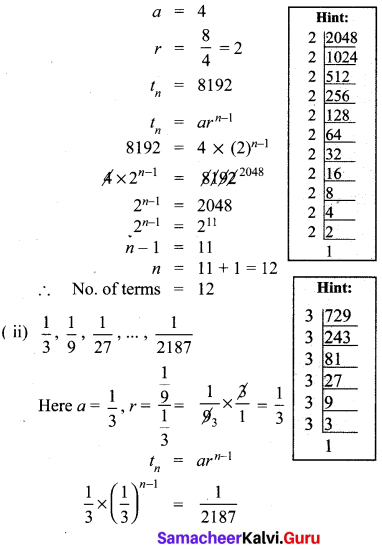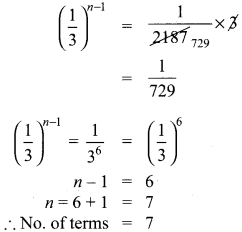Ex 2.7 Class 10 Question 6.
In a G.P. the 9th term is 32805 and 6th term is 1215. Find the 12th term.
Solution:
In a G.P
tn = arn-1
t9 = 32805
t6 = 1215
t12 = ?
Let
t9 = ar8 = 32805 ………(1)
t6 = ar5 = 1215 ………. (2)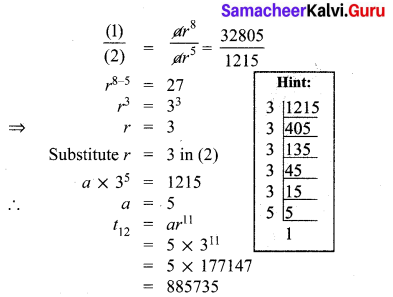10th Maths 2.7 Exercise Question 7.
Find the 10th term of a G.P. whose 8th term is 768 and the common ratio is 2.
Here r = 2, t8 = 768
t8 = 768 (tn = arn-1)
a. r8-1 = 768
ar7 = 768 …..(1)
10th term of a G.P. = a.r 10-1
= ar9
= (ar7) × (r2)
= 768 × 22 (from 1)
= 768 × 4 = 3072
∴ 10th term of a G.P. = 3072

10th Math Exercise 2.7 Solution Question 8.
If a, b, c are in A.P. then show that 3a,3b,3c are in G.P.
Solution:
If a, b, c are in A.P
t2 – t1 = t3 – t2
b – a = c – b
2b = c + a
To prove that 3a, 3b, 3c are in G.P
⇒ 32b = 3c+a + a [Raising the power both sides]
⇒ 3b.3b = 3c.3a
$$\Rightarrow \frac{3^{b}}{3^{a}}=\frac{3^{c}}{3^{b}}$$
$$\Rightarrow \frac{t_{2}}{t_{1}}=\frac{t_{3}}{t_{1}}$$
⇒ Common ratio is same for 3a,3b,3c
⇒ 3a, 3b, 3c forms a G.P
∴ Hence it is proved .

Exercise 2.7 Class 10 Maths Solution Question 9.
In a G.P. the product of three consecutive terms is 27 and the sum of the product of two terms taken at a time is $$\frac { 57 }{ 2 }$$. Find the three terms.
Solution:
Let the three consecutive terms in a G.P are $$\frac { a }{ r }$$, a, ar.
Their Product = $$\frac { a }{ r }$$ × a × ar = 27
a3 = 27 = 33
a = 3
Sum of the product of terms taken two at a time is $$\frac { 57 }{ 2 }$$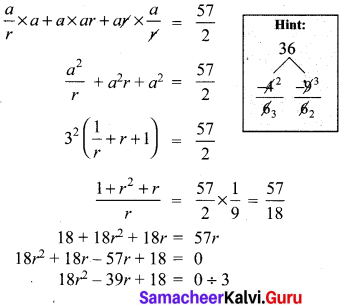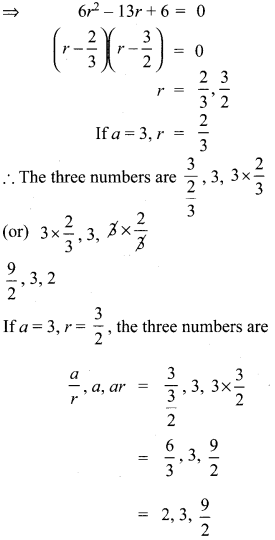10th Maths 2.7 Question 10.
A man joined a company as Assistant Manager. The company gave him a starting salary of ₹60,000 and agreed to increase his salary 5% annually. What will be his salary after 5 years?
Solution:
Starting salary = ₹ 60,000
Increase per year = 5%
∴ At the end of 1 year the increase
= 60,0,00 × $$\frac { 5 }{ 100 }$$
₹ 3000
∴ At the end of first year his salary
= ₹ 60,000 + 3000
I year salary = ₹ 63,000
II Year increase = 63000 × $$\frac { 5 }{ 100 }$$
At the end of II year, salary
= 63000 + 3150
= ₹ 66150
III Year increase = 66150 × $$\frac { 5 }{ 100 }$$
= 3307.50
At the end of III year, salary = 66150 + 3307.50
= ₹ 69457.50
IV year increase = 69457.50 × $$\frac { 5 }{ 100 }$$
= ₹ 3472.87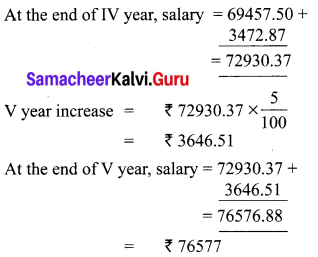2.7 Maths Class 10 Question 11.
Sivamani is attending an interview for a job and the company gave two offers to him. Offer A: ₹ 20,000 to start with followed by a guaranteed annual increase of 3% for the first 5 years.
Offer B: ₹ 22,000 to start with followed by a guaranteed annual increase of 3% for the first 5 years.
What is his salary in the 4th year with respect to the offers A and B?
Solution:
Offer A
Starting salary ₹ 20,000
Annual increase 6%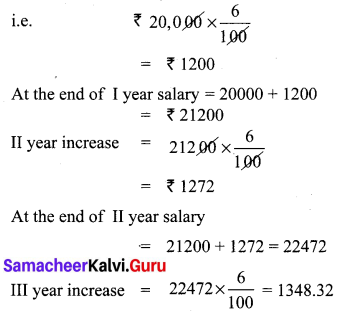At the end of
III year ,salary = 22472 + 1348 = 23820
∴ IV year salary = ₹ 23820
Offer B
Starting salary = ₹ 22,000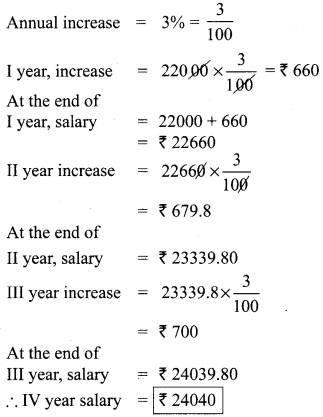Salary as per Option A = ₹ 23820
Salary as per Option B = ₹ 24040
∴ Option B is better.

Ex2.7 Class 10 Question 12.
If a, b, c are three consecutive terms of an A.P. and x, y, z are three consecutive terms of a G.P. then prove that xb-c × yc-a × za-b = 1.
Solution:
a, b, c are three consecutive terms of an AP.
∴ Let a, b, c be a, a + d, a + 2d respectively ………… (1)
x, y, z are three consecutive terms of a GP.
∴ Assume x, y, z as x, x.r, x.r2 respectively ……… (2)
PT : xb-c , yc-a , za-b = 1
Substituting (1) and (2) in LHS, we get
LHS = xa+d-a-2d × (xr)a+2d-a × (xr2)a-a-d
= (x)-d . (xr)2d (xr2)-d
= $$\frac{1}{x^{d}}$$ × x2d . r2d × $$\frac{1}{x^{d} r^{2 d}}$$ = 1 = RHS